# Random walks#

Random walks are an essential building blocks in stochastics but also in Xenakis music and are used in macro- and microcomposition. Before we use it in a sonic environment we want to explore them in a formalistic and programming manner. We start by taking a look at the classical symmteric random walk followed by the random walk with boundaries. This will then allow us to understand the notion of 2nd order random walk which uses Xenakis in his Stochastic Synthesis which can be heard in such compositions as Gendy 3 (1991).

The programming language for this chapter will be Python as some plotting is easier in this language.

The chapter is based on [Sch15] and [Luq06].

import numpy as np
import matplotlib.pyplot as plt
import matplotlib as mpl
import pandas as pd
from IPython.display import display, Audio

mpl.rcParams['figure.figsize'] = (15, 5)

np.random.seed(42)


## Formal introduction of random walks#

For now we restrict ourselves to symmetric random walks, which mean they are as likely to go up as they are likely to go down, and therefore both options have a chance of $$50\%$$.

We therefore start by defining our steps $$(Y_n)_{n \in \mathbb{N}}$$ of our random walk $$X = (X_n)_{n \in \mathbb{N}_0}$$. Each $$(Y_n)_{n \in \mathbb{N}}$$ must be independent of each other and must obey the same distribution

$\mathbb{P}[Y_n = 1] = 0.5 = \mathbb{P}[Y_n = -1]$

We define that our random walk starts at $$0$$, so $$X_0 = 0$$ and each following step is defined by

$X_n = \sum_{i=1}^n Y_i$

As we now have the formal definition we can try this out in practice by programming it in Python.

# 10 numbers of either -1 or 1
y = np.random.choice([-1, 1], size=10)
y

array([-1,  1, -1, -1, -1,  1, -1, -1, -1,  1])

# sum them up using cumulative sum
x = np.cumsum(y)
x

array([-1,  0, -1, -2, -3, -2, -3, -4, -5, -4])


The intuition behind random walks comes a bit clearer when we begin to plot them.

def random_walk(steps: int):
y = np.random.choice([-1, 1], size=steps)
x = np.cumsum(y)
return x

steps = 1000
x = random_walk(steps)
plt.plot(x)
plt.axhline(0.0, c='r')
plt.xlim(left=0)
plt.title(f"Random walk with {steps} steps");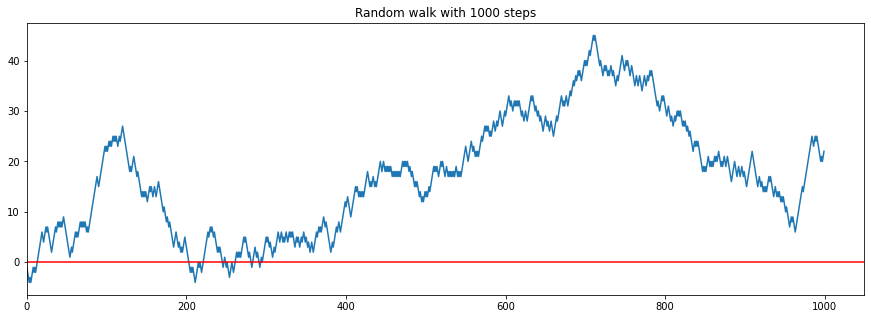Each successive point either goes up or down by $$1$$.

We can also run multiple random walks next to each other.

steps = 1000
for i in range(20):
x = random_walk(steps)
plt.plot(x)
plt.axhline(0.0, c='r')
plt.xlim(left=0)
plt.title(f"Random walk with {steps} steps");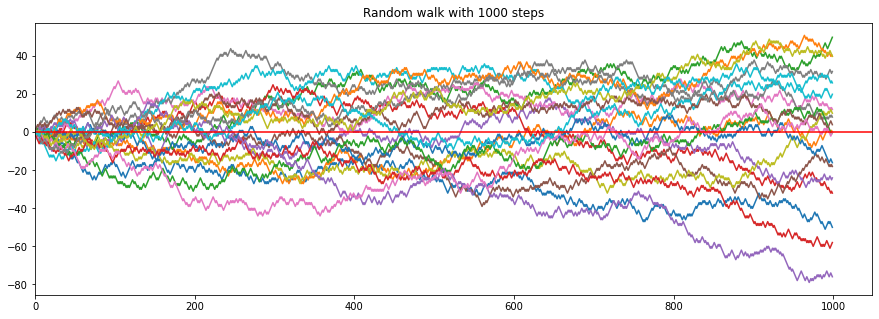Although we go $$10'000$$ steps in vertical movement the lateral movement rarely exceeds 100 - althogh it is also possible that it is reaches $$10'000$$ (everytime it goes to the up-direction), but this is highely unlikely - we can even calculate this.

As all $$(Y_n)_{n \in \mathbb{N}}$$ are independent we can simply multiplying every time the possibility of going up which is $$\frac{1}{2}$$, therefore

$\mathbb{P}[X_{10'000}=10'000] = \underbrace{\frac{1}{2} \cdot \frac{1}{2} \cdots \frac{1}{2}}_{10'000\text{ times}} = \frac{1}{2^{10'000}} \approx 5.0 \times 10^{-3011}$

which is a number with over 3000 zeros after the dot.

We note that $$\mathbb{P}[X_{10'000}=10'001]=0.0$$ because it is impossible to sum up $$10'000$$ possible $$1$$ s to $$10'001$$ when starting from $$X_0 = 0$$.

## Random walk with barriers#

We can also restrict the domain of random walk $$(X_n)_{n \in \mathbb{N}}$$ to be between $$-l < (X_n)_{n \in \mathbb{N}} < l$$ for a given $$l \in \mathbb{N}$$. This seemingly small changes makes the formalism a bit more complicated because if we hit the barrier $$l$$ our decission is not random anymore as we are not allowed to exceed the boundary and therefore have to go into the opposite direction of the barrier. Therefore our random variables $$(Y_n)_{n \in \mathbb{N}}$$ are not independent anymore as they now depend on each other. A way to solve this is by defining random variables not as above but via markov chains.

We define the event space for our markov chain via $$E = \mathbb{Z}$$. For $$p\in(0, 1)$$ is the transitin matrix $$A$$ with a state $$i \in E$$ defined via

$a_{i, i+1} = p = 1-a_{i, i-1}$

and elsewhere $$0$$. For $$p=0.5$$ the markov chain is equal to the single dimensional random walk as defined above. Therefore is the random walk only a special case of a markov chain. The transition matrix $$A$$ looks therefore like

$\begin{split} A = \begin{bmatrix} \ddots & \vdots & \vdots & \vdots & \vdots & \vdots & \ddots \\ \dots & a_{2, -2} & a_{2, -1} & a_{2, 0} & a_{2, 1} & a_{2, 2} & \dots \\ \dots & a_{1, -2} & a_{1, -1} & a_{1, 0} & a_{1, 1} & a_{1, 2} & \dots \\ \dots & a_{0, -2} & a_{0, -1} & a_{0, 0} & a_{0, 1} & a_{0, 2} & \dots \\ \dots & a_{-1, -2} & a_{-1, -1} & a_{-1, 0} & a_{-1, 1} & a_{-1, 2} & \dots \\ \dots & a_{-2, -2} & a_{-2, -1} & a_{-2, 0} & a_{-2, 1} & a_{-2, 2} & \dots \\ \ddots & \vdots & \vdots & \vdots & \vdots & \vdots & \ddots \\ \end{bmatrix} = \begin{bmatrix} \ddots & \vdots & \vdots & \vdots & \vdots & \vdots & \ddots \\ \dots & 0 & 0 & 0 & 0.5 & 0 & \dots \\ \dots & 0 & 0 & 0.5 & 0 & 0.5 & \dots \\ \dots & 0 & 0.5 & 0 & 0.5 & 0 & \dots \\ \dots & 0.5 & 0 & 0.5 & 0 & 0 & \dots \\ \dots & 0 & 0.5 & 0 & 0 & 0 & \dots \\ \ddots & \vdots & \vdots & \vdots & \vdots & \vdots & \ddots \\ \end{bmatrix} \end{split}$

But the notion of the event space allows us to change the modify the transition matrix as we can now implement a limit. For a given $$l \in \mathbb{Z}$$ we modify the transitin matrix $$A$$ with

$\begin{split} a_{i, i+1} = \begin{cases} 0~&\text{if}~i=l\\ 1~&\text{if}~i=-l\\ p~&\text{else}\\ \end{cases} ~~~~~~~~~~ a_{i, i-1} = \begin{cases} 0~&\text{if}~i=-l\\ 1~&\text{if}~i=l\\ p~&\text{else}\\ \end{cases} \end{split}$

We will only implement an imperative way to implement such a limited random walk, but it could be also implemented as a markov chain.

def random_walk_boundary(steps: int, limit: int):
y = []
x_cur = 0
step_size = 1

for _ in range(steps):
# check if we hit a barrier
if np.abs(x_cur) + step_size > limit:
step = np.sign(x_cur) * (-1 * step_size)
else:
step = np.random.choice([step_size, -1*step_size])
x_cur += step
y.append(step)

x = np.cumsum(y)
return x

steps = 1000
limit = 10

x = random_walk_boundary(steps, limit)
plt.plot(x)
plt.axhline(0.0, c='r')
for l in [limit, -1*limit]:
plt.axhline(l, c='g')
plt.xlim(left=0)
plt.title(f"Random walk with {steps} steps and limit {limit}");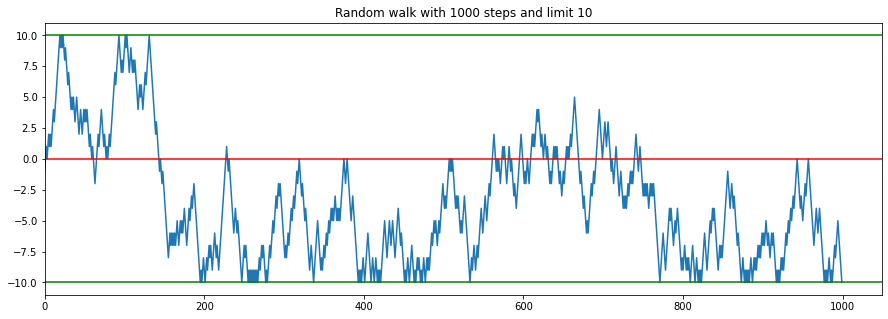## Second order random walks#

Before we define second random order walks we will take a look at why Xenakis introduced those and why traditional random walks are not sufficient. For this we will take a look at the distribution (we will count how often each stage of the random walk is passed) of two very longe traditional random walks, one limited, the other not.

x = []
for _ in range(100):
x.append(random_walk(100000))
x = np.concatenate(x)
pd.Series(x).hist(bins=100)
plt.title("Distribution of a non-limited random walk");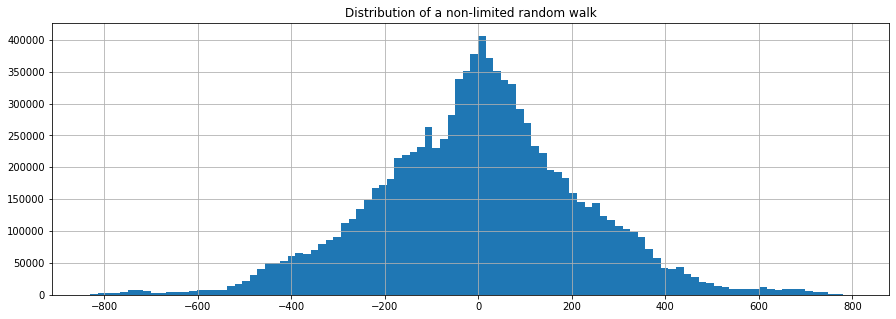We see that the traditional random walk fluctuates around $$0$$ most of the time and looks like a gaussian distribution. This phonomenon is described by the central limit theorem.

x = []
for _ in range(100):
x.append(random_walk_boundary(1000, limit=20))
x = np.concatenate(x)
pd.Series(x).hist(bins=20)
plt.title("Distribution of a limited random walk")

Text(0.5, 1.0, 'Distribution of a limited random walk')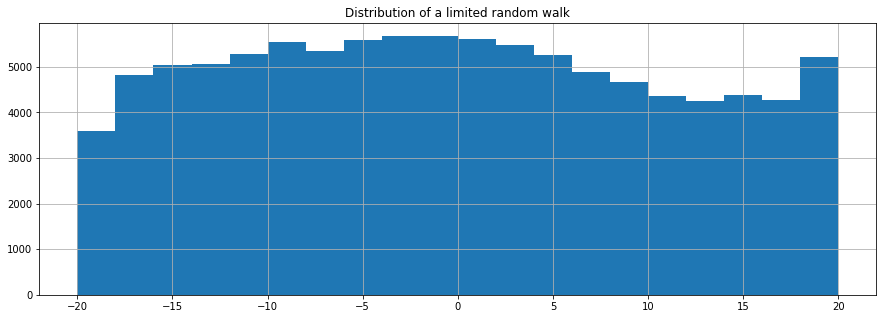The limited random walk would eventually yield the same result from above if we run it enough times, but as its barriers reflect we would have to do this much longer.

Why do we take a look at the distributions??

We only get noise from it, see.

sample_rate = 48000
# 16 bits divided by 2 (b/c we need to cover + and -)
amp = ((2**16)/2)*0.4

x = random_walk(sample_rate*4)
# scale x to be between -1 and 1
x = x/np.max(np.abs(x))
display(Audio(data=(x*amp).astype(np.int16) , rate=sample_rate))


This generates only 4 seconds of noise.

x = random_walk_boundary(sample_rate*4, limit=200)
# scale x to be between -1 and 1
x = x/np.max(np.abs(x))
display(Audio(data=(x*amp).astype(np.int16) , rate=sample_rate))